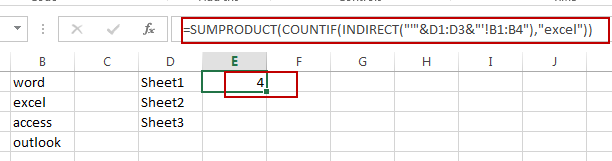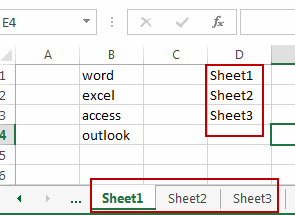# Countif across Multiple Worksheets

This post will guide you count a value across multiple worksheets in excel. How do I use COUNTIF function to get the number of occurrence of a value from multiple worksheets in Excel. How to count the number of values from multiple worksheets in Excel. How to count based on a single criteria across multiple sheets.

## Countif across Multiple Worksheets

Assuming that you have three worksheets in your current workbook, and you want to count the number of a specified text string “excel” in same range B1:B4 across those three worksheets. How to achieve it. You can do the following steps:

#1 type all three worksheet names in one single column.#2 type the following formula into a blank cell, and press Enter key.

`=SUMPRODUCT(COUNTIF(INDIRECT("'"&D1:D3&"'!B1:B4"),"excel"))`

#3 the number of text string “excel” in the same range B1:B4 among three worksheet (sheet1, sheet2 and sheet3) has been calculated in cell E1.### Related Functions

• Excel SUMPRODUCT function
The Excel SUMPRODUCT function multiplies corresponding components in the given one or more arrays or ranges, and returns the sum of those products.The syntax of the SUMPRODUCT function is as below:= SUMPRODUCT (array1,[array2],…)…
• Excel INDIRECT function
The Excel INDIRECT function returns the cell reference based on a text string, such as: type the text string “A2” in B1 cell, it just a text string, so you can use INDIRECT function to convert text string as cell reference….
• Excel COUNTIF function
The Excel COUNTIF function will count the number of cells in a range that meet a given criteria. This function can be used to count the different kinds of cells with number, date, text values, blank, non-blanks, or containing specific characters.etc.= COUNTIF (range, criteria)…